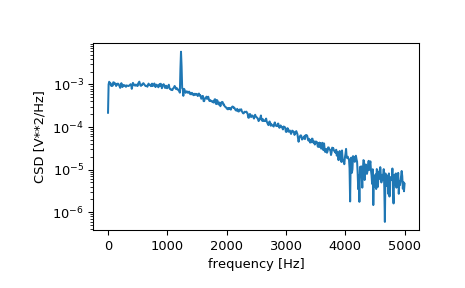# scipy.signal.csd¶

scipy.signal.csd(x, y, fs=1.0, window='hann', nperseg=None, noverlap=None, nfft=None, detrend='constant', return_onesided=True, scaling='density', axis=-1, average='mean')[source]

Estimate the cross power spectral density, Pxy, using Welch’s method.

Parameters
xarray_like

Time series of measurement values

yarray_like

Time series of measurement values

fsfloat, optional

Sampling frequency of the x and y time series. Defaults to 1.0.

windowstr or tuple or array_like, optional

Desired window to use. If window is a string or tuple, it is passed to get_window to generate the window values, which are DFT-even by default. See get_window for a list of windows and required parameters. If window is array_like it will be used directly as the window and its length must be nperseg. Defaults to a Hann window.

npersegint, optional

Length of each segment. Defaults to None, but if window is str or tuple, is set to 256, and if window is array_like, is set to the length of the window.

noverlap: int, optional

Number of points to overlap between segments. If None, noverlap = nperseg // 2. Defaults to None.

nfftint, optional

Length of the FFT used, if a zero padded FFT is desired. If None, the FFT length is nperseg. Defaults to None.

detrendstr or function or False, optional

Specifies how to detrend each segment. If detrend is a string, it is passed as the type argument to the detrend function. If it is a function, it takes a segment and returns a detrended segment. If detrend is False, no detrending is done. Defaults to ‘constant’.

return_onesidedbool, optional

If True, return a one-sided spectrum for real data. If False return a two-sided spectrum. Note that for complex data, a two-sided spectrum is always returned.

scaling{ ‘density’, ‘spectrum’ }, optional

Selects between computing the cross spectral density (‘density’) where Pxy has units of V**2/Hz and computing the cross spectrum (‘spectrum’) where Pxy has units of V**2, if x and y are measured in V and fs is measured in Hz. Defaults to ‘density’

axisint, optional

Axis along which the CSD is computed for both inputs; the default is over the last axis (i.e. axis=-1).

average{ ‘mean’, ‘median’ }, optional

Method to use when averaging periodograms. Defaults to ‘mean’.

New in version 1.2.0.

Returns
fndarray

Array of sample frequencies.

Pxyndarray

Cross spectral density or cross power spectrum of x,y.

periodogram

Simple, optionally modified periodogram

lombscargle

Lomb-Scargle periodogram for unevenly sampled data

welch

Power spectral density by Welch’s method. [Equivalent to csd(x,x)]

coherence

Magnitude squared coherence by Welch’s method.

Notes

By convention, Pxy is computed with the conjugate FFT of X multiplied by the FFT of Y.

If the input series differ in length, the shorter series will be zero-padded to match.

An appropriate amount of overlap will depend on the choice of window and on your requirements. For the default Hann window an overlap of 50% is a reasonable trade off between accurately estimating the signal power, while not over counting any of the data. Narrower windows may require a larger overlap.

New in version 0.16.0.

References

1

P. Welch, “The use of the fast Fourier transform for the estimation of power spectra: A method based on time averaging over short, modified periodograms”, IEEE Trans. Audio Electroacoust. vol. 15, pp. 70-73, 1967.

2

Rabiner, Lawrence R., and B. Gold. “Theory and Application of Digital Signal Processing” Prentice-Hall, pp. 414-419, 1975

Examples

>>> from scipy import signal
>>> import matplotlib.pyplot as plt


Generate two test signals with some common features.

>>> fs = 10e3
>>> N = 1e5
>>> amp = 20
>>> freq = 1234.0
>>> noise_power = 0.001 * fs / 2
>>> time = np.arange(N) / fs
>>> b, a = signal.butter(2, 0.25, 'low')
>>> x = np.random.normal(scale=np.sqrt(noise_power), size=time.shape)
>>> y = signal.lfilter(b, a, x)
>>> x += amp*np.sin(2*np.pi*freq*time)
>>> y += np.random.normal(scale=0.1*np.sqrt(noise_power), size=time.shape)


Compute and plot the magnitude of the cross spectral density.

>>> f, Pxy = signal.csd(x, y, fs, nperseg=1024)
>>> plt.semilogy(f, np.abs(Pxy))
>>> plt.xlabel('frequency [Hz]')
>>> plt.ylabel('CSD [V**2/Hz]')
>>> plt.show()#### Previous topic

scipy.signal.welch

#### Next topic

scipy.signal.coherence